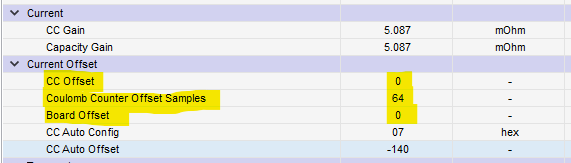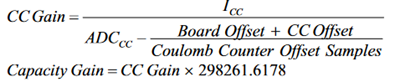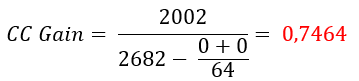If you have a related question, please click the "Ask a related question" button in the top right corner. The newly created question will be automatically linked to this question.

# BQ28Z610-R1: Calculation of CC Gain / Capacity Gain

Part Number: BQ28Z610-R1
Other Parts Discussed in Thread: BQSTUDIO

I have to calibrate the bq28z610-R1 with an external tool. No bqStudio. I follow the stept from TRM and stuck with the formula for CC Gain.

Icc = 2002mA

ADCcc = 0xF5 86 (got from 0xF081 and MACData() AA 3E 81 F0 7A 01 86 F5 01 46 49 4A 00 00 ....)

with 2 comp is ADCcc =  2682 DEC = 0xA7A

If I fill the values from data memory ...... in the formula from TRM.....I get an unexpeted result. It should be around 5.000.

Is there a hidden trick behind it again?

• Hello Martin,

The floating point you calculated (0.7464) seems correct. That CC gain value would work if you programmed it into your gauge. The reason why you don't get 5mohm is because the units are different. The value you see in BQstudio takes the number you calculated (0.7464) and a conversion factor (3.714528) and does a division to make it mohms for the user. So what you would see in BQstudio is 3.714528/0.7464 = 4.977 mohm. So, 0.7464 is a perfectly fine result to put into the CC gain when programming, its just that BQstudio is automatically converting units for ease of use.

thanks,

Alex M.

• Hello Alex,

thanks for this enlightenment. So i have to convert the 0.7464 floating point into an 4 byte HEX (see in TRM BQ28Z610-R1 13.1.3) to transmit it in little endian to the bq.

So my calclation result for 0.7465 is 0x39F9

I write AA 3E 06 40 F9 39 with verification AA 60 87 06 and my CC Gain in Data Memory switchs to 5.107 and not 4.977. So my current measurment is not correct (1950mA rather than 2002mA). Have I done something wrong?

Second question:

I guess for capacity gain has an internal value too?

Capacity Gain= 0,7465 × 298261,6178= 222622,47

So my calclation result for 222622.47 is 0x7586 and after write it to bq i got 5.092 in Data Memory. So there is something wrong, too.

• Hello Martin,

Can you try writing 0x3F3F1AA0 to CC gain and see if that works for you? I see you are using a 16-bit floating point with 0x39F9 but the TRM lays out a 32-bit process. I may be misunderstanding so let me know if I'm wrong, but see if it works using the longer one. Also, you can iterate if it still isn't as precise as you would like afterwards.

thanks,

Alex M.

• With the declaration "IEEE754 Single Precision 4-byte format in Little Endian byte" I expect a floating point of 16-bit, but your suggestion with 8-bytes seams to work.

I wrote W: AA 3E 06 40 A0 1A 3F 3F and got the CC Gain 4.976  in Data Memory and that's correct.

To complete the second question about Capacity Gain:

Capacity Gain = 0.7465 × 298261.6178 = 222652.3 = 0x48596F13

W: AA 3E 0A 40 13 6F 59 48
W: AA 60 92 08

Leads to the result that in Capacity Gain there is also 4.976. That's correct.